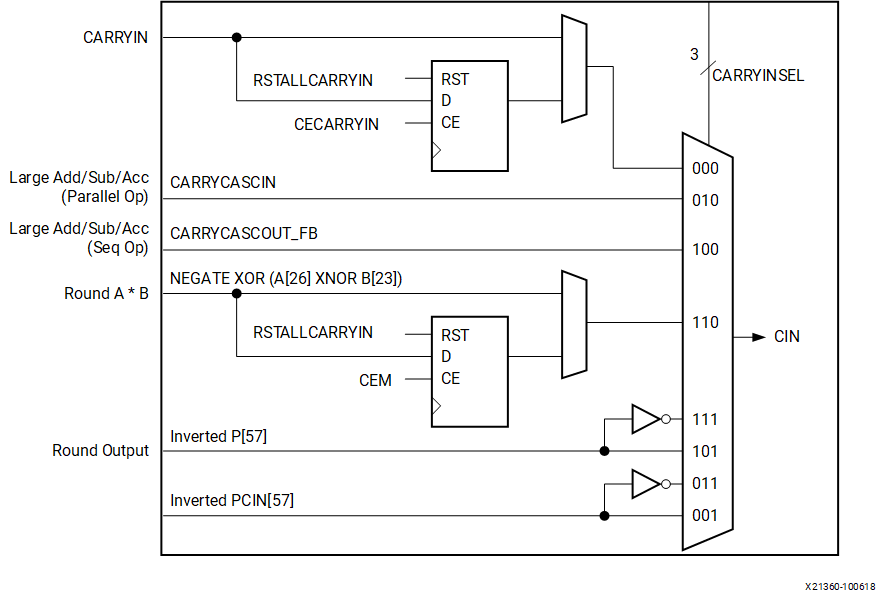# Carry Input Logic

## Versal ACAP DSP Engine Architecture Manual (AM004)

Document ID
AM004
Release Date
2022-09-11
Revision
1.2.1 English

The carry input logic result is a function of a 3-bit CARRYINSEL signal. The inputs to the carry input logic appear in the following figure. Carry inputs used to form results for adders and subtracters are always in the critical path. High performance is achieved by implementing this logic in silicon. The possible carry inputs to the carry logic are gathered prior to the outputs of the W, X, Y, and Z multiplexers. CARRYIN has no dependency on the OPMODE selection.

Figure 1. CARRYINSEL Port LogicThe figure above shows eight inputs selected by the 3-bit CARRYINSEL control. The first input, CARRYIN (CARRYINSEL set to binary `000`), is driven from general logic. This option allows implementation of a carry function based on user logic. CARRYIN can be optionally registered. The next input, (CARRYINSEL is equal to binary `010`) is the CARRYCASCIN input from an adjacent DSP58. The third input (CARRYINSEL is equal to binary `100`) is the CARRYCASCOUT from the same DSP58, fed back to itself.

The fourth input (CARRYINSEL is equal to binary `110`) is the complement of the sign bit of the product (NEGATE XOR (A XNOR B)) for symmetrically rounding multiplier outputs towards infinity. This signal can be optionally registered to match the MREG pipeline delay. The fifth and sixth inputs (CARRYINSEL is equal to binary `111` and `101`) selects the true or inverted P output MSB P for symmetrical rounding.

The seventh and eight inputs (CARRYINSEL is equal to binary `011` and `001`) selects the true or inverted cascaded P input MSB PCIN for symmetrically rounding the cascaded P input.

The following table lists the possible values of the three carry input select bits (CARRYINSEL) and the resulting carry inputs or sources.

Table 1. CARRYINSEL Control Carry Source
CARRYINSEL Select Notes
2 1 0
`0` `0` `0` CARRYIN General interconnect
`0` `0` `1` ~PCIN Rounding PCIN (round towards infinity)
`0` `1` `0` CARRYCASCIN Larger add/sub/acc (parallel operation)
`0` `1` `1` PCIN Rounding PCIN (round towards zero)
`1` `0` `0` CARRYCASCOUT_FB (CARRYCASCOUT in the same DSP58) For larger add/sub/acc (sequential operation through internal feedback). Requires PREG = `1`
`1` `0` `1` ~P Rounding P (round towards infinity). Requires PREG = `1`
`1` `1` `0` NEGATE XOR (A XNOR B) Symmetric rounding of A x B towards infinity. This value is invalid in CINT18 mode
`1` `1` `1` P For rounding P (round towards zero). Requires PREG = `1`